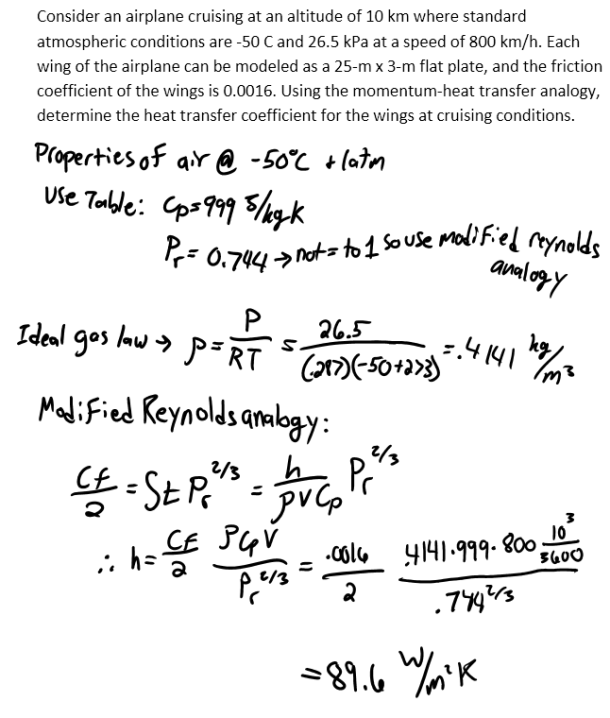heat and mass transfer problems engineering equations heat and mass transfer school homework engineering heat and mass transfer formulas heat and mass transfer solutions to heat and mass transfer problems full solution engineering problem solution heat and mass transfer math problems engineering equations heat and mass transfer school homework engineering solutions to heat and mass transfer formulas heat problems mass problem solutions to transfer problems full solution heat and mass transfer
heat and mass transfer problems engineering equations heat and mass transfer school homework engineering heat and mass transfer formulas heat and mass transfer solutions to heat and mass transfer problems full solution engineering problem solution heat and mass transfer math problems engineering equations heat and mass transfer school homework engineering solutions to heat and mass transfer formulas heat problems mass problem solutions to transfer problems full solution heat and mass transfer
Highalphabet Home Page heat and mass transfer problem solutions Heat and Mass Transfer Page
Consider an airplane cruising at an altitude of 10 km where standard atmospheric conditions are -50 C and 26.5 kPa at a speed of 800 km/h. Each wing of the airplane can be modeled as a 25-m x 3-m flat plate, and the friction coefficient of the wings is 0.0016. Using the momentum-heat transfer analogy, determine the heat transfer coefficient for the wings at cruising conditions.Consider an airplane cruising at an altitude of 10 km where standard atmospheric conditions are -50 C and 26.5 kPa at a speed of 800 km/h. Each wing of the airplane can be modeled as a 25-m x 3-m flat plate, and the friction coefficient of the wings is 0.0016. Using the momentum-heat transfer analogy, determine the heat transfer coefficient for the wings at cruising conditions.